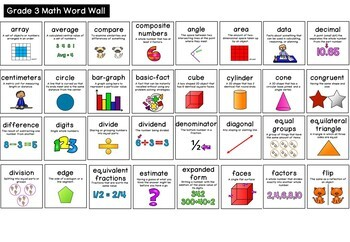# Math Word Wall (Grade 3)Subject
Grade Levels
Resource Type
Product Rating
File Type

Compressed Zip File

Be sure that you have an application to open this file type before downloading and/or purchasing.

5 MB
Share
Product Description

Math Word Wall (Grade 3)

This pack includes 102 posters for a Math Word Wall in your Grade 3 class. Each word has a definition and a picture. This is a great way to help your students with their Maths Vocabulary. I have also included both US and AUS/UK Spelling in two separate files so that they can be used in any country. Each poster can be printed in either A5 or A4 size. (see preview for detailed view)

The following words have been included:

angle, area, array, average, bar graph, basic fact, data, decimal, decimal point, denominator, diagonal, difference, digits, divide, dividend, division, divisor, edge, equal groups, equally likely equilateral triangle, equivalent amounts, equivalent fractions, estimate, expanded form, faces factors ,flip, fraction, gram (g), hexagon, hour (h), hundred thousands, hundredths, improper fraction, intersecting lines, irregular polygon, is greater than, is less than, is not equal to, isosceles triangle, key, kilogram, kilometer, leap year, line, line of symmetry, line segment, litre, mass, map, metre, millimeter, minute, mixed numeral, mud-map, multiple, multiplication, net, numerator, octagon, order, ordered pair, ordinal numbers, outcome, volume, palindrome, parallel lines, parallelogram, pentagon, perimeter, perpendicular, pictograph, plane figure, polygon, prime number, prism, probability, product, prime number, pyramid, quadrilateral, quarter, quotient, ray, rectangle, rectangular prism, reflect, reflection, regroup, regular polygon, remainder, right angle, right triangle, rotate, round, rounding, scale, side, sphere square square number sum survey symmetry table tally tally marks temperature ten thousands tenths thousands triangle turn unit fraction value vertex (vertices)

Total Pages
N/A
Answer Key
N/A
Teaching Duration
N/A
Report this ResourceSign Up